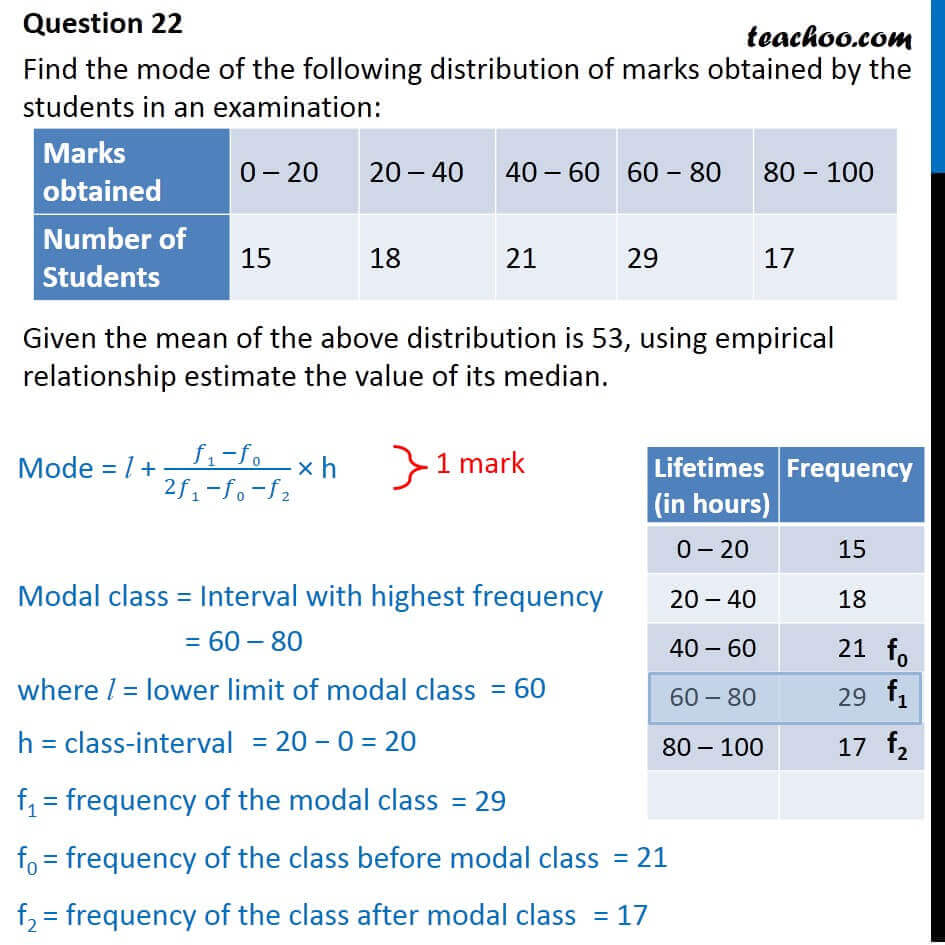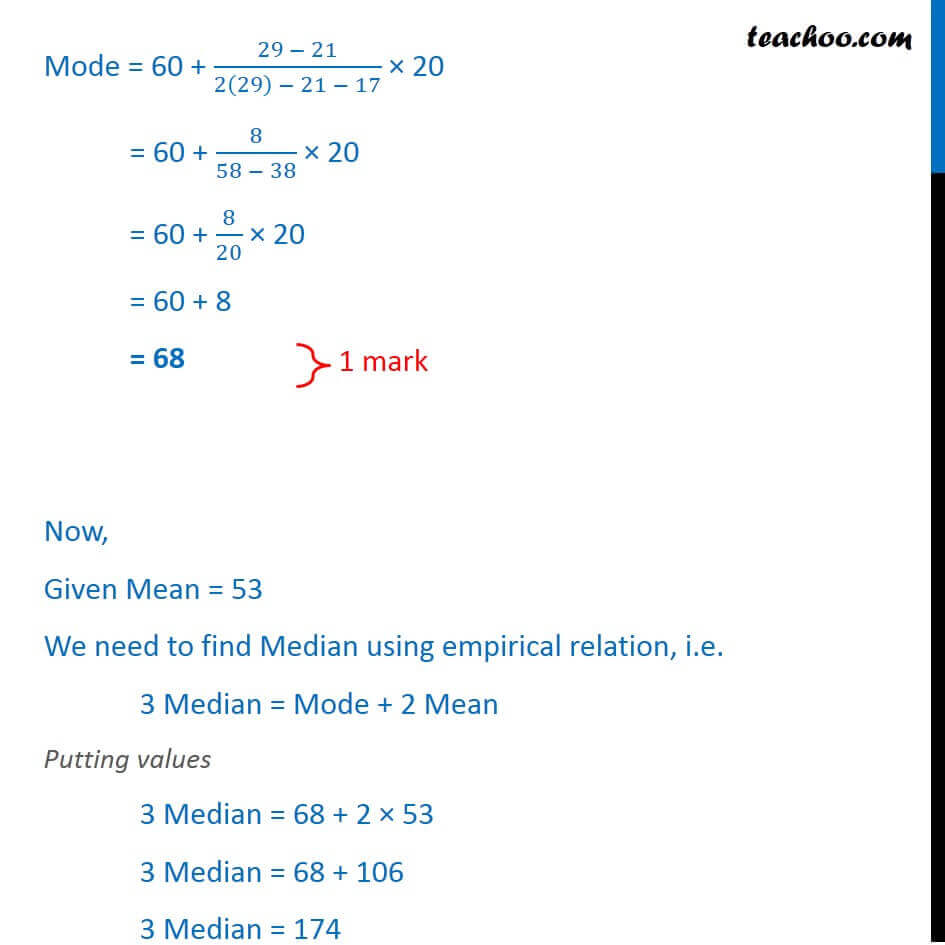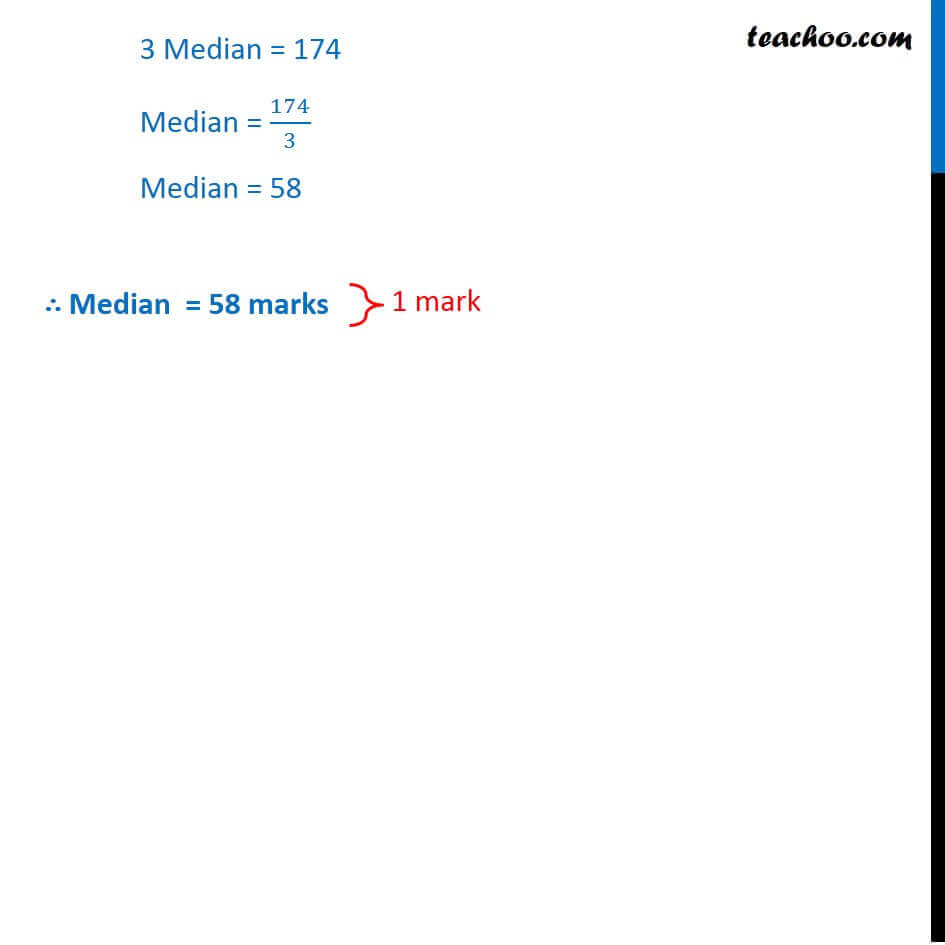CBSE Class 10 Sample Paper for 2018 Boards

Class 10
Solutions of Sample Papers for Class 10 Boards

### Find the mode of the following distribution of marks obtained by the students in an examination:

 Marks obtained 0 – 20 20 – 40 40 – 60 60 − 80 80 − 100 Number of Students 15 18 21 29 17

### Given the mean of the above distribution is 53, using empirical relationship estimate the value of its median.

This is a question of CBSE Sample Paper - Class 10 - 2017/18.Learn in your speed, with individual attention - Teachoo Maths 1-on-1 Class

### Transcript

Question 22 Find the mode of the following distribution of marks obtained by the students in an examination: Marks obtained 0 – 20 20 – 40 40 – 60 60 − 80 80 − 100 Number of Students 15 18 21 29 17 Given the mean of the above distribution is 53, using empirical relationship estimate the value of its median. Mode = l + (𝑓1 −𝑓0)/(2𝑓1 −𝑓0 −𝑓2) × h Modal class = Interval with highest frequency = 60 – 80 where l = lower limit of modal class h = class-interval f1 = frequency of the modal class f0 = frequency of the class before modal class f2 = frequency of the class after modal class Lifetimes (in hours) Frequency 0 – 20 15 20 – 40 18 40 – 60 21 60 – 80 29 80 – 100 17